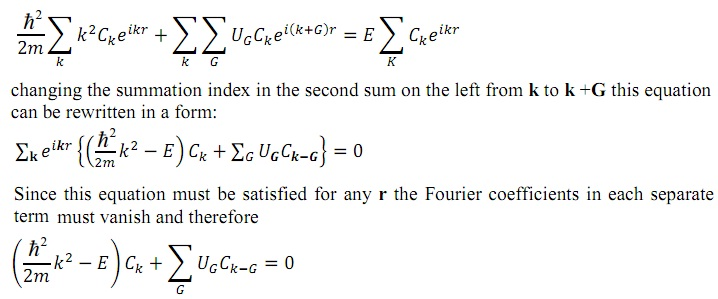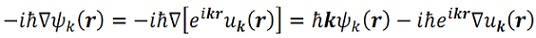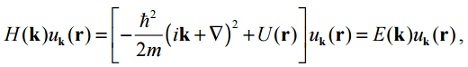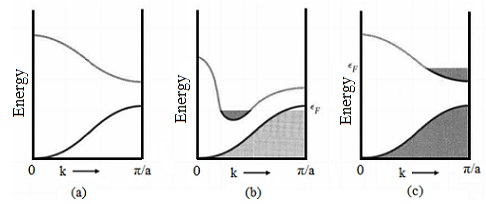#### Energy Band Theory, Physics tutorial

Introduction:

The free electron model provides us a good insight into numerous properties of metals, like the heat capacity, thermal conductivity and electrical conductivity. Though, this model fails to help us by other significant properties. For illustration, it doesn't predict the difference between the metals, semiconductors and insulators. It doesn't illustrate the occurrence of positive values of the Hall coefficient. As well the relation between the conduction electrons in the metal and the number of valence electrons in free atoms is not for all time correct. We require a more accurate theory that would be capable to answer such questions.

Definition:

Energy band is the range of energies possessed via electrons in a solid.

Energy band:

This is customary to imagine the existence of bands on an energy scale of band structure scheme, according to which, the energy bands for the most tightly bound electrons lie at the bottom, followed via the band of the second most tightly bound electrons, and so forth, till we reach the top of the set of entirely full energy bands. The top of the band of the set is termed as the valence band. Next higher energy band is termed to as the conduction band that may be fully empty. The characteristic energy which separate the occupied from empty states is termed as Fermi energy EF and is characterized via Fermi level existing between the conduction band and the valence band. The two bands are separated through energy gap Eg, stated by:

Eg = Ec - Ev

Here EC and Ev are correspondingly the energy of the bottom of the conduction band and the top of the valence band. The Eg value for a semiconductor is usually of the order of 1 eV and that for an insulator is 5 eV based on the relative positions of conduction and valence bands, metals might be categorized into two groups. In one, valence band is entirely full and conduction band is partly filled, illustration: Na, 2p (valence) band is completely full and conduction (3s) band is half filled. In the other, conduction and valence bands overlap one other. For illustration: Mg (1s2, 2s2, 2p6, 3s2), 3s2 (valence) and 3p (conduction) bands overlap in energy.

Periodic Potential:

The potential seen via an electron due to the nucleus of an isolated atom of valence 'z' is -ze2/r, here 'e' is the electronic charge and 'r' the nucleus - electron distance. Though, the atom in a perfect crystal are set in a regular periodic array, thus, we are led to consider the problem of an electron in a potential U(r) having the periodicity of the under-lying Bravais lattice that is,

U(r) = U(r + T)

Here, 'T' is a lattice vector. Qualitatively, a usual crystalline potential may be expected to encompass a form shown in the figure given below, resembling the individual atomic potentials as the ion is approached closely and flattening off in the area between ions.As the scale of periodicity of the potential U (~ 10-8 cm) is the size of a typical de Broglie wavelength of the electron, it is necessary to use quantum mechanics in accounting for the effect of periodicity on the electronic motion. Therefore we consider the Hamiltonian.

H(r) = - [(h/2π)2/2m]∇2 + U(r)

By using the equation U(r) = U(r + T) in the above equation leads to:

H(r + T) = H(r)

This illustrates that the Hamiltonian as well consists of the lattice periodicity. Therefore, to predict the physical properties of the crystal, one must resolve the given Schrodinger equation for a single electron:

H(Ψ) = [{(h/2π)2/2m}∇2 + U(r)] Ψ(r) = EΨ(r)

in which ψ(r) is a wave function for one electron. Independent electrons that obey a one electron Schrodinger equation (above equation) having a periodic potential, are termed as Bloch electrons, in disparity to 'free electrons', to which the Bloch electrons reduce if the periodic potential is identically (0) zero.

Now we illustrate general properties of the solution of the Schrodinger equation (above equation) taking into account periodicity of the effective potential [U(r) = U(r + T)] and describe the major properties of Bloch electrons that follow from this solution. We represent the solution as an expansion over the plain waves.

Ψ(r) = Σk ckeikr

This expansion in the Fourier series is a natural generalization of the free-electron solution for a zero potential. The summary in the above equation is carrying out over all k vectors that are permitted through the periodic boundary conditions. According to such conditions the wave function of the above equation must satisfy:

Ψ(x, y, z) = Ψ(x + L, y, z) = Ψ(x, y + L, z) = Ψ(x, y, z + L)

So that,

Kx = 2πnx/L; Ky = 2πny/L; Kz = 2πnz/L

Here nx, ny, nz are the positive or negative integers. It will be noted that in general ψ (r) is not periodic in the lattice translation vectors. On other hand, according to equation [U(r) = U(r + T)] the potential energy is periodic, that is, it is invariant beneath a crystal lattice translation. Thus, its plane wave expansion will only have plane waves having the periodicity of the lattice. Thus, only reciprocal lattice vectors are left in the Fourier expansion for the potential:

U(r) = ΣG UGeiGr

Here the Fourier coefficients UG are associated to U(r) by:

UG = (1/VC)∫celle-iGr U(r)dr

Here, VC is the volume of the unit cell. It is simple to see that certainly the potential energy symbolized by [U(r) = ΣG UGeiGr] is periodic in the lattice:

U (r + T) = ΣG UGeiG(r+T) = eiGT ΣG UGeiGr = U(r)

Here, the last equation comes from the statement of the reciprocal lattice vectors eiGT = 1. The values of Fourier components UG for actual crystal potentials tend to reduce rapidly by increasing magnitude of G. For illustration: for a Coulomb potential UG reduces as 1/G2. It will be noted that as the potential energy is real, the Fourier components must satisfy U-G = U*G

We now replace [Ψ(r) = Σk ckeikr] and [U(r) = ΣG UGeiGr] in equation H(Ψ) = [{(h/2π)2/2m}∇2 + U(r)] Ψ(r) = EΨ(r) and get:This is the set of linear equations for the coefficients Ck. Such equations are nothing however restatement of the original Schrodinger equation in the momentum space, simplified via the fact that the potential is periodic. This set of equations doesn't look very pleasant as, in principle, an infinite number of coefficients must be determined. Though, a careful examination of the above equation leads to significant effects.

At first, we see that for a fixed value of k the set of above equations couples only those coefficients, whose wave vectors vary from k via a reciprocal lattice vector. In one-dimensional case these are k, k ± 2π/a, k ± 4π/a and so forth. We can thus suppose that the k vector belongs to the first Brillouin zone. The original problem is decoupled to 'N' independent problems (that is, 'N' is the total number of atoms in the lattice): for each allowed value of 'k' in the first Brillouin zone. Each such problem consists of solutions that are superposition of plane waves having only the wave vector k and wave vectors differing from k through the reciprocal lattice vector.

Placing this information back to the expansion Ψ(r) = Σk ckeikr of the wave function ψ (r), we notice that the wave function will be of the form:

Ψk(r) = ΣG Ck-G ei(K-G)r

Here the summation is carried out over the reciprocal lattice vectors and we introduced index k for the wave function. We can reorganize this so that:

Ψk(r) = eiKr ΣG Ck-G eiGr

Or, Ψk(r) = eiKr uk(r)

Here uk(r) = uk(r + T) is the periodic function which is stated by:

uk(r) = ΣG Ck-G eiGr

The equation Ψk(r) = eiKr uk(r) is termed as Bloch theorem that plays a significant role in the electronic band structure theory. Now we illustrate a number of significant conclusions that follow from the Bloch theorem.

1) Bloch's theorem proposes a wave vector 'k' that plays the similar fundamental role in the general problem of motion in the periodic potential that the free electron wave vector 'k' plays in the free-electron theory. It will be noted that however the free electron wave vector is simply p/(h/2π), here 'p' is the momentum of the electron, in the Bloch case 'k' is not proportional to the electronic momentum. This is clear on general grounds, as the Hamiltonian doesn't have complete translational invariance in the presence of the non-constant potential and thus its Eigen states will not be simultaneous Eigen states of the momentum operator. This conclusion is proved by the fact that the momentum operator, p = - i(h/2π)∇, when acting on ωk(r) provides:That is not, in general, just a constant time ωk(r); that is, ωk(r) is not a momentum Eigen state. Nonetheless, in numerous ways (h/2π) k is a natural extension of p to the case of the periodic potential. This is termed as the crystal momentum or quasi momentum of the electron, to emphasize this similarity, however one must not be misled by the name into thinking that (h/2π) k is a momentum.

2) The wave vector 'k' coming out in Bloch's theorem can for all time be confined to the first Brillouin zone (or to any other well-situated primitive cell of the reciprocal lattice). This is because any k' not in the first Brillouin zone can be represented as:

K' = K + G

Here, 'G' is the reciprocal lattice vector and 'k' does lie in the first zone. As eiGT = 1 for any reciprocal lattice vector, if the Bloch form the equation Ψk(r) = eiKr uk(r) holds for k', it will as well hold for 'k'. An illustration is given below for a nearly free electron model.

The energy 'E' of free electrons that is plotted versus k in the first part of figure below represents a curve in the familiar parabolic shape. The second part of the figure represents the result of translations. Segments of the parabola of first part are cut at the edges of different zones, and are translated by multiples of G = 2π/a in order to make sure that the energy is similar at any two equivalent points. The third part of the figure shows the shape of the energy spectrum whenever we confine our consideration to the first Brillouin zone only. The kind of representation utilized in the third part of figure is termed to as the reduced-zone scheme. As it specifies all the required information, it is the one we shall determine most convenient. The representation of the first part of figure, termed as the extended-zone scheme is convenient whenever we wish to emphasize the close connection between the crystalline and a free electron. Second part of figure uses the periodic-zone scheme, and is at times helpful in topological considerations comprising the 'k' space. All such representations are strictly equivalent; the use of any specific one is dictated by convenience, and not through any intrinsic benefits it has over the others.3) The significant effect of the Bloch theorem is the appearance of the energy bands. All solutions to the Schrodinger equation have the Bloch form Ψk(r) = eiKr uK(r) here 'k' is fixed and uk(r) consists of the periodicity of the Bravais lattice. Replacing this into the Schrodinger equation, we determine that uK(r) is found out by the eigenvalue problem.With boundary condition:

uk(r) = uk(r + T)

Due to the periodic boundary condition we can regard the above equation as an eigenvalue problem limited to a single primitive cell of the crystal. As the eigenvalue problem is set in a fixed finite volume, we suppose on general grounds to determine an infinite family of solutions having discretely spaced Eigen values that we label by the band index 'n'. The Bloch function can thus be represented by Ψnk(r) that points out that each and every value of the band index 'n' and the vector 'k' specifies an electron state, or orbital having energy En(k).

It will be noted that in terms of the eigenvalue problem specified through the above two equation, the wave vector 'k' appears only as the parameter in the Hamiltonian H(k). We thus anticipate each of the energy levels, for given 'k', to vary continuously as k varies. In this manner we arrive at an explanation of the levels of an electron in a periodic potential in terms of a family of continuous functions En(k).For each 'n', the set of electronic levels specified by En(k) is termed as an energy band. The information contained in such functions for different 'n' and 'k' is termed to as the band structure of the solid.

4) Number of states in a band.

The number of orbitals in a band in the first Brillouin zone is equivalent to the number of unit cells 'N' in the crystal. This is much similar as the statement made in connection by the number of lattice vibrational modes, and is proved in a way, by appealing to the boundary conditions.

Consider first the one-dimensional case. The allowed values of 'k' make a uniform mesh whose unit spacing is 2π/L. The number of states within the first zone, whose length is 2π/a, is thus equivalent to (2π/a)/(2π/L) = L/a = N, here 'N' is the number of unit cells, in agreement by the assertion made earlier.

An identical argument might be employed to establish the validity of the statement in two and three dimensional lattices.

This has been represented that each and every band consists of 'N' states within the first zone. As each such state can accommodate at most two electrons, of opposite spins, in accordance by the Pauli Exclusion Principle, it follows that the maximum number of electrons that might occupy a single band is 2N.

5) Now we represent that an electron in a level specified by band index 'n' and wave vector k has a non-vanishing mean velocity, represented by:This is a remarkable fact. It states that there are stationary levels for an electron in the periodic potential in which, despite the interaction of the electron having the fixed lattice of ions, it moves forever devoid of any degradation of its mean velocity. This is in striking dissimilarity to the idea of Drude that collisions were simply encounters among the electron and a static ion.

Metals and Insulators:

Solids are categorized into two main classes: metals and insulators. A metal or a conductor is a solid in which the electric current flows under the application of the electric field. By dissimilarity, application of an electric field generates no electric current in the insulator. There is a simple principle for differentiating between the two classes on the base of the band structure. When the valence electrons precisely fill one or more bands, leaving others empty, the crystal will be the insulator. The external electric field will not cause current flow in the insulator. Given that a filled band is separated through energy gap from the next higher band, there is no continuous manner to change the net momentum of the electrons if each and every accessible state is filled. Nothing alters if the field is applied.Fig: Occupied states and band structures giving (a) The insulator (b) Metal or a semimetal due to band overlap and (c) A metal due to electron concentration.

On the contrary, if the valence band is not fully filled the solid is a metal. In a metal there are empty states available above the Fermi level such as in a free electron gas. The application of an external electric field outcome in the current flow.

This is possible to find out whether a solid is a metal of an insulator by considering the number of valence electrons. The crystal can be an insulator only if the number of valence electrons in a primitive cell of the crystal is an even integer. This is due to the reason that each and every band can accommodate only two electrons per primitive cell. For illustration: diamond consists of two atoms of valence four, in such a way that there are eight valence electrons per primitive cell. The band gap in diamond is 7eV and this crystal is a good insulator.

Though, if a crystal consists of an even number of valence electrons per primitive cell, it is not essentially an insulator. This might happen that the bands overlap in energy. Whenever the bands overlap in energy, then rather than one filled band giving an insulator, we can encompass two partially filled bands giving a metal (as shown in the second part of figure). For illustration, the divalent metals, like Mg or Zn, have two valence electrons per cell. Though, they are metals, however a poor ones - their conductivity is small.

When this overlap is extremely small, we deal by the semimetals. The best recognized illustration of a semimetal is bismuth (Bi).

If the number of valence electrons per cell is odd, then the solid is a metal. For illustration: the alkali metals and the noble metals encompass one valence electron per primitive cell, in such a way that they have to be metals.

The alkaline earth metals encompass two valence electrons per primitive cell; they could be insulators, however the bands overlap in energy to give metals, however not very good metals. Diamond, silicon and germanium each encompass two atoms of valence four, in such a way that there are eight valence electrons per primitive cell; the bands don't overlap, and the pure crystals are insulators at absolute zero.

There are substances that fall in an intermediate position among metals and insulators. Whenever the gap between the valence band and the band immediately above it is small, then the electrons are readily excitable thermally from the former to the latter band. Both the bands become only partly filled and both contribute to the electric condition. Such a substance is termed as a semiconductor. Illustrations are Si and Ge, in which the gaps are around 1 and 0.7 eV, correspondingly.

Generally speaking, a substance behaves as a semiconductor at room temperature if the gap is less than 2 eV. The conductivity of a usual semiconductor is extremely small compared to that of a metal; however it is still many orders of magnitude bigger than that of an insulator. This is justifiable, thus, to categorize semiconductors as a new class of substance, however they are, strictly speaking, insulators at extremely low temperatures.

Tutorsglobe: A way to secure high grade in your curriculum (Online Tutoring)

Expand your confidence, grow study skills and improve your grades.

Since 2009, Tutorsglobe has proactively helped millions of students to get better grades in school, college or university and score well in competitive tests with live, one-on-one online tutoring.

Using an advanced developed tutoring system providing little or no wait time, the students are connected on-demand with a tutor at www.tutorsglobe.com. Students work one-on-one, in real-time with a tutor, communicating and studying using a virtual whiteboard technology.  Scientific and mathematical notation, symbols, geometric figures, graphing and freehand drawing can be rendered quickly and easily in the advanced whiteboard.

Free to know our price and packages for online physics tutoring. Chat with us or submit request at info@tutorsglobe.com